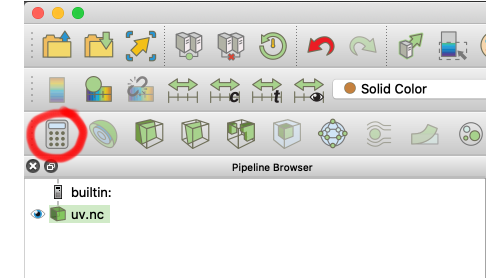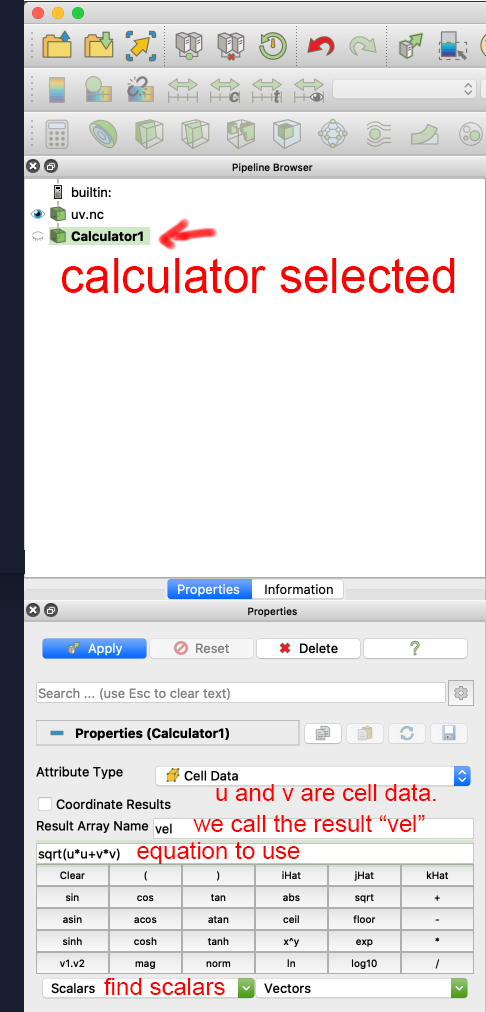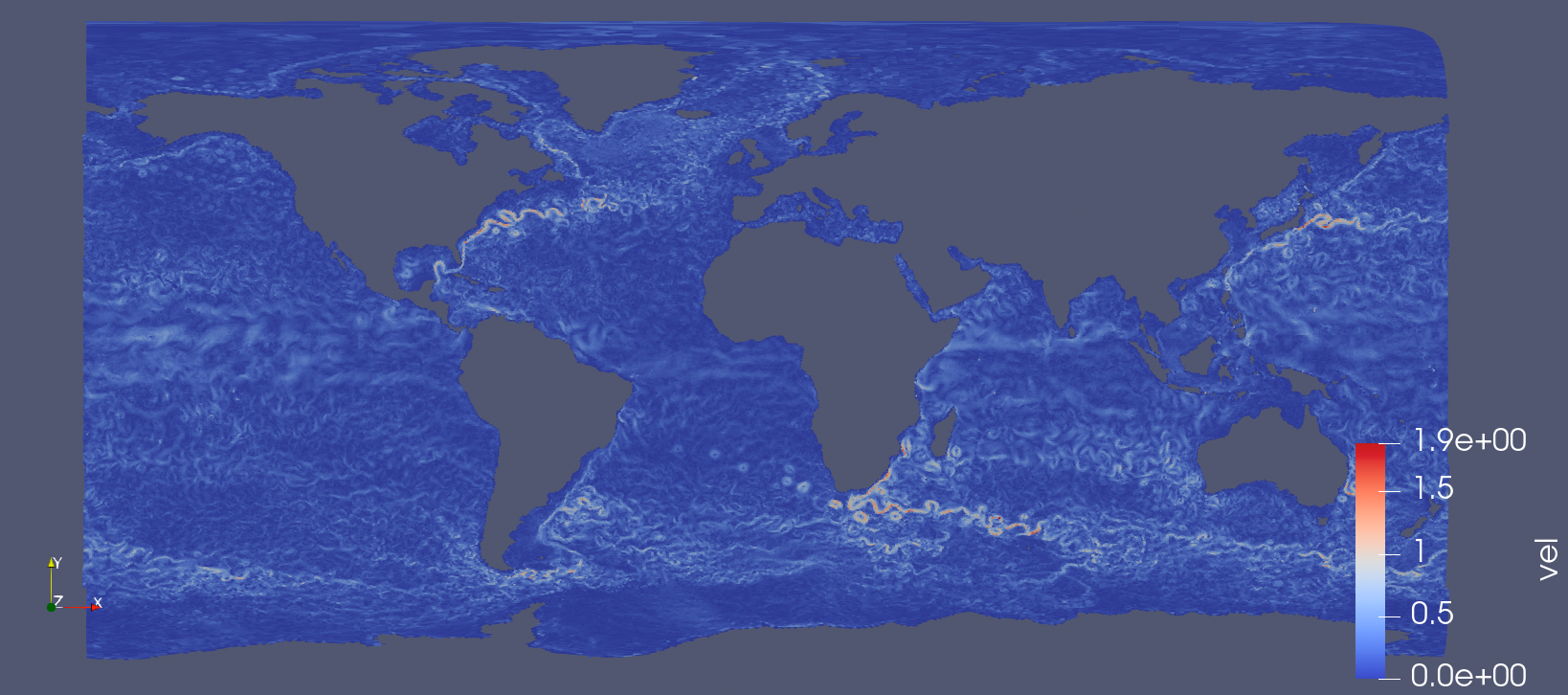# Apply a calculator to convert u and v into speed#

Note

This document is part of a series teaching how to Create an image of sea surface speeds

Here we assume you alred loaded 2D ocean data

Now we want to apply a “Calculator” filter to compute the absolute speed from the velocity. Choose Filters->Common->Calculator, or use the calculator symbol just above the pipeline.In the calculator, you can chose the data type to operate on (“cell data” is correct here), give your result variable a sensible name – we chose `vel` here – `speed` would have been correct…

Naming your output immediately is useful, as color maps are associated with these names, so “Result” can lead to conflicts and hassle once you have a second calculator.

In the next field, you can define the equation that is computed. We want to use:

```sqrt (u*u+v*v)
```

You can also use the drop-down menus at the bottom to get access to the variables available (sorted by scalars and vector quantities).The result should look similar to this:With the speed computed, you can Chose a different colormap

Alternatively, you can Rescale the colormap to a custom range